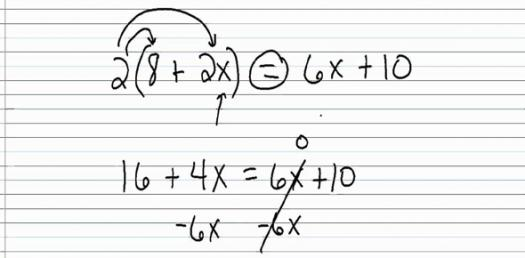# Problem Solving For Algebra

10 Questions | Total Attempts: 54SettingsIt is a quiz for algebraic expressions.

• 1.
Three runners  averaged "y" minutes. Express their total running time.
• A.

3x

• B.

Y+3

• C.

3y

• 2.
What is the difference of Anelo's weight (x) and Thelma's weight (y)?
• A.

2x-y

• B.

X-y

• C.

X+y

• 3.
Express the total weight of Sassy the dog (x) and Felix the cat (y).
• A.

X+y

• B.

X+2y

• C.

X-y

• 4.
What if the number (x) of children was increased by six, and then they had to share twenty dollars? How much would each get?
• A.

20(x+6)

• B.

20/(x+6)

• C.

20(6x)

• 5.
The length of a ballfield is 50 yards more than its width "y."Express the length of the field in terms of its width y.
• A.

50y

• B.

50y+x

• C.

Y+50

• 6.
Express the number (x)  of oranges increased by two.
• A.

X+2

• B.

2x

• C.

2x+2

• 7.
What is 8 less than y?
• A.

8-y

• B.

Y-8

• C.

X-8

• 8.
What is y multiplied by 12?
• A.

10+2y

• B.

12/(y)

• C.

12y

• 9.
Five students rent an apartment for Php "x" /month.What will each have to pay?
• A.

5x/5

• B.

X/5

• C.

5/x

• 10.
What is the quotient of y and 3?
• A.

Y/3

• B.

3/y

• C.

3(y)

Related TopicsBack to top